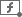Application:

If you select a range of cells, MyOffice Spreadsheet automatically calculates 5 of the most commonly used functions and displays the results in the Status bar:

SUM: Calculates the sum of all numbers in the selected range.

AVERAGE: Calculates the average value of all numbers in the selected range.

MIN: Displays the smallest value among all the numbers in the selected range.

MAX: Displays the highest value among all the numbers in the selected range.

COUNTA: Displays the number of values in the selected range. Numerical and text values are considered.

To configure the list of available functions, follow these steps:

1. In the Status bar, click.

2. In the opened list, check the boxes of the functions that will be displayed in the Status bar.